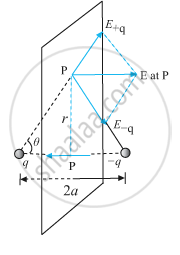# Derive an Expression for the Electric Field Due to a Dipole of Dipole Moment → P at a Point on Its Perpendicular Bisector. - Physics

Derive an expression for the electric field due to a dipole of dipole moment vec"p" at a point on its perpendicular bisector.

#### SolutionThe magnitudes of the electric field due to the two charges +q and −q are given by,

E_(+q) = ("q")/(4πε_0) (1)/("r"^2 + a^2)     .....(i)

E_(-q) = ("q")/(4πε_0) (1)/("r"^2 + a^2)     .....(i)

∴ E_(+q) = E_(-q)

The directions of E+q and E−q are as shown in the figure. The components normal to the dipole axis cancel away. The components along the dipole axis add up.
Total electric field

E = -(E_+q + E_-q) cos theta  hat"p"   ... ["negative sign shows that field is opposite to"  hat"p"]

E = -(2qa)/(4πε_0("r"^2 + a^2)^(3/2)) hat"p"    ....(iii)

At large distances (r >> a), this reduces to

E = -(2qa)/(4πε_0"r"^3)hat"p"             ....(iv)

vec"p" = q xx  vec"2a"hat"p"

E = (-vec"p")/(4πε_0"r"^3)    ...(r>>a).

Is there an error in this question or solution?
2018-2019 (March) 55/1/3

Share